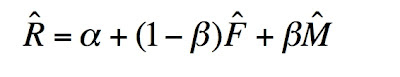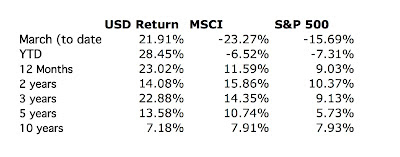## Sunday, March 18, 2007

### Expected Returns

Following on from my previous post, one of the uses that this alpha/beta analysis can be put to is estimating your future expected rate of return. You just need to plug in the expected risk free rate and benchmark rate of return into the following formula:The "hats" indicated "estimated value". This formula ignores the uncertainty in the estimates of the coefficients. You could do a Monte Carlo simulation to find the expected distribution of future returns. But for now I will assume that the coefficients are known with certainty. Based on my MSCI analysis I come up with:

alpha = 11.26%
beta = 0.72

For the S&P 500 I get:

alpha = 16.07%
beta = 0.58

(see what I mean by the MSCI being a steeper hurdle?). Now if I assume that F = 4.98% (its current value) and M = 10.5% I come up with expected rates of return of 20.2% and 20.1% based on the two benchmarks. Of course, you can assume a lower rate for M if you want. How do these compare with my actual returns?All these rates are annualized. For the last 12 months and the last 3 years my annualized rates of return are 23%. However, 2005 was a bad year and so for the last two years the mean is just 14%. The MSCI achieved 11.6%, 14.4%, and 15.9% for the same periods. So the index was a bit above trend in those years and my own results for 1 or 3 years are also above trend. The S&P 500 has been at or below trend, however. Over the last 5 years I averaged 13.6% compared to 10.7% and 5.8%. My alpha has been increasing over time so this makes sense. Over a ten year horizon I underperformed the indices.

Let's assume that the 20% rate makes sense, then what does it imply? 20% of my \$215k in non-retirement accounts is \$43,000 roughly the US average salary. It is enough pre-tax income to cover my current expenses. I could "retire" now. The quotation marks mean that I would become a full-time active investor and trader. My retirement accounts would continue to grow at a rapid rate. Real retirement could happen at a later date if I wanted it. Of course, I would like to have a huge margin of safety so that if the rate of return is lower or my expenses rise rapidly I wouldn't run out of non-retirement money. The bottom line is though that all non-retirement saving I do from now on is increasing that margin of safety.